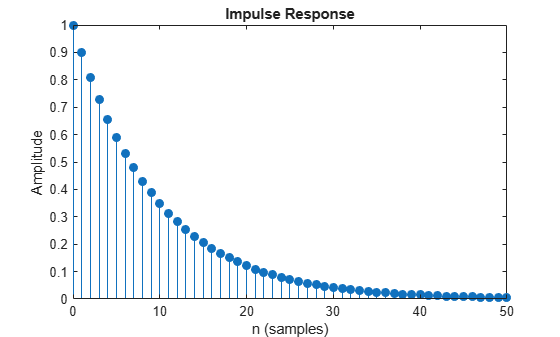# Impulse Response

The impulse response of a digital filter is the output arising from the unit impulse sequence defined as

`$\delta \left(n\right)=\left\{\genfrac{}{}{0}{}{1,\phantom{\rule{0.2777777777777778em}{0ex}}\phantom{\rule{0.2777777777777778em}{0ex}}n=0,}{0,\phantom{\rule{0.2777777777777778em}{0ex}}\phantom{\rule{0.2777777777777778em}{0ex}}n\ne 0.}$`

You can generate an impulse sequence a number of ways; one straightforward way is

`imp = [1; zeros(49,1)];`

The impulse response of the simple filter with $b=1$ and $a=\left[1\phantom{\rule{0.16666666666666666em}{0ex}}-0.9\right]$ is $h\left(n\right)=0.{9}^{n}$, which decays exponentially.

```b = 1; a = [1 -0.9]; h = filter(b,a,imp); stem(0:49,h)```A simple way to display the impulse response is with the Filter Visualization Tool, `fvtool`.

`fvtool(b,a)`Click the Impulse Response button, $\left[↑\right]$, on the toolbar, select Analysis > Impulse Response from the menu, or type the following code to obtain the exponential decay of the single-pole system.

`fvtool(b,a,'Analysis','impulse')`